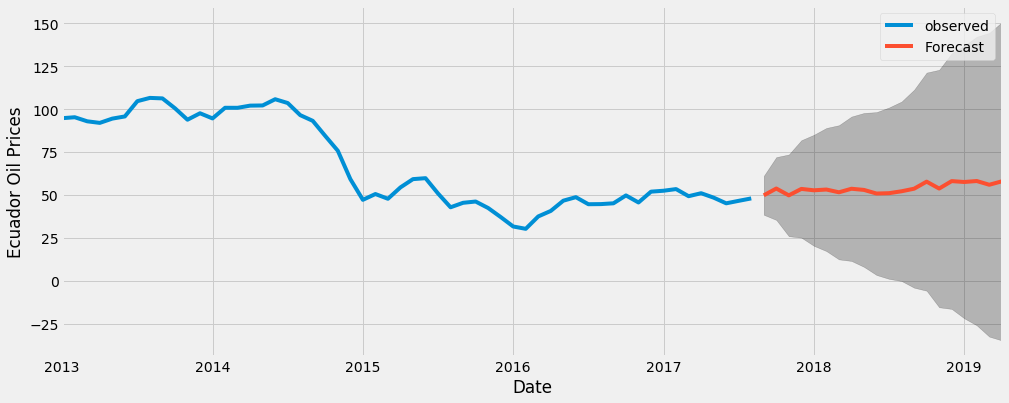Francisca Dias

The only thing that's predictable, it seems, is oil's unpredictability.

## Introduction¶

I agree with the statement above, that the only thing that is predictable is oil's price unpredictability.

Oil prices have significant impact on Ecuador's economy and it would be nice to see what the model has to tell us.

I am applying ARIMA since this is a time series data set. Oil prices range from 2013 till 2017.

I will try to explain as much as I can all steps I have taken in this analysis.

This dataset can be found here.

## Libraries¶

In :
import warnings
warnings.filterwarnings('ignore')
import itertools
import pandas as pd
import numpy as np
import statsmodels.api as sm
import matplotlib.pyplot as plt
plt.style.use('fivethirtyeight')

In :
oil = pd.read_csv("oil_ecuador.csv")

Out:
date dcoilwtico
0 2013-01-01 NaN
1 2013-01-02 93.14
2 2013-01-03 92.97
In :
oil.tail(3)

Out:
date dcoilwtico
1215 2017-08-29 46.46
1216 2017-08-30 45.96
1217 2017-08-31 47.26
In :
# convert to datetime
oil['date'] =  pd.to_datetime(oil['date'])

In :
oil = oil.rename(columns={'dcoilwtico': 'oilprice'})

In :
# set date as index
oil.set_index('date', inplace=True)

In :
# Daily data can be difficult to work with since it’s a short amount of time, so I will use monthly averages instead.
oil = oil['oilprice'].resample('MS').mean()

In :
plt.title("Ecuador's oil price Evolution")
plt.xlabel("Date")
plt.ylabel("Oil Price")
oil.plot(figsize=(15, 6))
plt.show()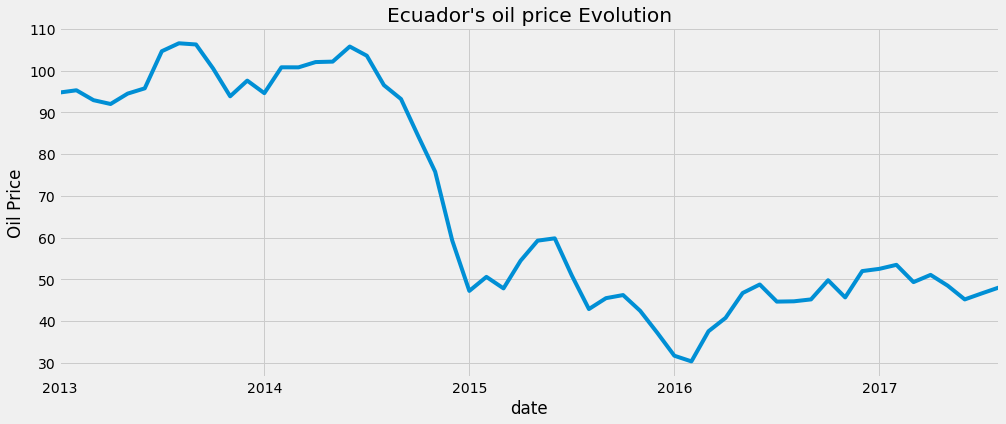There are patterns that can be noticed by looking at the graphic.

The time series has a modest seasonality pattern, where the oil price is higher in the middle of each year. But this my be insignificant.

And there is clearly an overall decreasing trend in oil prices.

## The ARIMA Time Series Model¶

For time series analysis, ARIMA model in one of the best methods that can be used to forecast.

It incorporates three parameters that take into account the properties of time series: seasonality, trend, and noise.

I will next describe how to automate the process of identifying the optimal set of parameters for the ARIMA model.

## Parameter Selection for the ARIMA Time Series Model¶

In order to do a correct parametrization of ARIMA models, I will make use of Python code to select the optimal parameter values for our ARIMA(p,d,q)(P,D,Q)s model.

I will use a "grid search" (or hyperparameter optimization) that will iterate over different combinations of parameters, and I will use the one that has the lowest AIC value.

The AIC (Akaike Information Criterion) measures how well a model fits the data while taking into account the overall complexity of the model.

In :
p = d = q = range(0, 2)

In :
pdq = list(itertools.product(p, d, q))


Please note that I am using s= 12 since this dataset was converted to a monthly basis.

Therefore I have 12 observations for each year.

In :
seasonal_pdq = [(x, x, x, 12) for x in list(itertools.product(p, d, q))]

In :
# 12 for yearly periods
print('Examples of parameter combinations for Seasonal ARIMA...')
print('SARIMAX: {} x {}'.format(pdq, seasonal_pdq))
print('SARIMAX: {} x {}'.format(pdq, seasonal_pdq))
print('SARIMAX: {} x {}'.format(pdq, seasonal_pdq))
print('SARIMAX: {} x {}'.format(pdq, seasonal_pdq))

Examples of parameter combinations for Seasonal ARIMA...
SARIMAX: (0, 0, 1) x (0, 0, 1, 12)
SARIMAX: (0, 0, 1) x (0, 1, 0, 12)
SARIMAX: (0, 1, 0) x (0, 1, 1, 12)
SARIMAX: (0, 1, 0) x (1, 0, 0, 12)

In :
warnings.filterwarnings("ignore") # specify to ignore warning messages

for param in pdq:
for param_seasonal in seasonal_pdq:
try:
mod = sm.tsa.statespace.SARIMAX(oil,
order=param,
seasonal_order=param_seasonal,
enforce_stationarity=False,
enforce_invertibility=False)

results = mod.fit()

print('ARIMA{}x{}12 - AIC:{}'.format(param, param_seasonal, results.aic))
except:
continue

ARIMA(0, 0, 0)x(0, 0, 1, 12)12 - AIC:461.52390966810447
ARIMA(0, 0, 0)x(0, 1, 1, 12)12 - AIC:1316.9438452419647
ARIMA(0, 0, 0)x(1, 0, 0, 12)12 - AIC:391.8333330411939
ARIMA(0, 0, 0)x(1, 0, 1, 12)12 - AIC:362.6489417811609
ARIMA(0, 0, 0)x(1, 1, 0, 12)12 - AIC:310.42368482499165
ARIMA(0, 0, 0)x(1, 1, 1, 12)12 - AIC:1259.8887606562503
ARIMA(0, 0, 1)x(0, 0, 0, 12)12 - AIC:549.7870279021369
ARIMA(0, 0, 1)x(0, 0, 1, 12)12 - AIC:399.9809162382947
ARIMA(0, 0, 1)x(0, 1, 0, 12)12 - AIC:348.9825632360937
ARIMA(0, 0, 1)x(0, 1, 1, 12)12 - AIC:1180.8893088730729
ARIMA(0, 0, 1)x(1, 0, 0, 12)12 - AIC:345.161308650509
ARIMA(0, 0, 1)x(1, 0, 1, 12)12 - AIC:312.30112406371086
ARIMA(0, 0, 1)x(1, 1, 0, 12)12 - AIC:272.11755822466966
ARIMA(0, 0, 1)x(1, 1, 1, 12)12 - AIC:2198.793937102382
ARIMA(0, 1, 0)x(0, 0, 1, 12)12 - AIC:258.8308508538914
ARIMA(0, 1, 0)x(0, 1, 1, 12)12 - AIC:1097.9162197416356
ARIMA(0, 1, 0)x(1, 0, 0, 12)12 - AIC:267.6490740736151
ARIMA(0, 1, 0)x(1, 0, 1, 12)12 - AIC:263.54213033625825
ARIMA(0, 1, 0)x(1, 1, 0, 12)12 - AIC:201.98362485469536
ARIMA(0, 1, 0)x(1, 1, 1, 12)12 - AIC:1231.4493589339554
ARIMA(0, 1, 1)x(0, 0, 0, 12)12 - AIC:323.00047085367345
ARIMA(0, 1, 1)x(0, 0, 1, 12)12 - AIC:252.48399825443389
ARIMA(0, 1, 1)x(0, 1, 0, 12)12 - AIC:273.18086385901296
ARIMA(0, 1, 1)x(0, 1, 1, 12)12 - AIC:1263.0929161437784
ARIMA(0, 1, 1)x(1, 0, 0, 12)12 - AIC:264.2340233825461
ARIMA(0, 1, 1)x(1, 0, 1, 12)12 - AIC:254.36172019084415
ARIMA(0, 1, 1)x(1, 1, 0, 12)12 - AIC:202.15034072286642
ARIMA(0, 1, 1)x(1, 1, 1, 12)12 - AIC:1236.8777605387147
ARIMA(1, 0, 0)x(0, 0, 0, 12)12 - AIC:337.4068485559228
ARIMA(1, 0, 0)x(0, 0, 1, 12)12 - AIC:264.1382838826483
ARIMA(1, 0, 0)x(0, 1, 0, 12)12 - AIC:288.25762077160385
ARIMA(1, 0, 0)x(0, 1, 1, 12)12 - AIC:1142.9066879660732
ARIMA(1, 0, 0)x(1, 0, 0, 12)12 - AIC:267.12825659685217
ARIMA(1, 0, 0)x(1, 0, 1, 12)12 - AIC:266.1091776782345
ARIMA(1, 0, 0)x(1, 1, 0, 12)12 - AIC:201.21746347196242
ARIMA(1, 0, 0)x(1, 1, 1, 12)12 - AIC:1136.4670835206196
ARIMA(1, 0, 1)x(0, 0, 0, 12)12 - AIC:328.4616694104135
ARIMA(1, 0, 1)x(0, 0, 1, 12)12 - AIC:256.2541891904837
ARIMA(1, 0, 1)x(0, 1, 0, 12)12 - AIC:279.79323574951076
ARIMA(1, 0, 1)x(0, 1, 1, 12)12 - AIC:1434.0935775329792
ARIMA(1, 0, 1)x(1, 0, 0, 12)12 - AIC:264.31017561662634
ARIMA(1, 0, 1)x(1, 0, 1, 12)12 - AIC:258.16259269412296
ARIMA(1, 0, 1)x(1, 1, 0, 12)12 - AIC:201.71243221702716
ARIMA(1, 0, 1)x(1, 1, 1, 12)12 - AIC:1427.8677867326037
ARIMA(1, 1, 0)x(0, 0, 0, 12)12 - AIC:326.0490537780237
ARIMA(1, 1, 0)x(0, 0, 1, 12)12 - AIC:254.33600352460633
ARIMA(1, 1, 0)x(0, 1, 0, 12)12 - AIC:277.44373367078504
ARIMA(1, 1, 0)x(0, 1, 1, 12)12 - AIC:1328.3898023844463
ARIMA(1, 1, 0)x(1, 0, 0, 12)12 - AIC:255.84369254279744
ARIMA(1, 1, 0)x(1, 0, 1, 12)12 - AIC:256.33576997150544
ARIMA(1, 1, 0)x(1, 1, 0, 12)12 - AIC:196.28174700310274
ARIMA(1, 1, 0)x(1, 1, 1, 12)12 - AIC:1301.238218839072
ARIMA(1, 1, 1)x(0, 0, 0, 12)12 - AIC:322.65556223258415
ARIMA(1, 1, 1)x(0, 0, 1, 12)12 - AIC:251.9327611308941
ARIMA(1, 1, 1)x(0, 1, 0, 12)12 - AIC:273.4908675830158
ARIMA(1, 1, 1)x(0, 1, 1, 12)12 - AIC:1268.1872307365327
ARIMA(1, 1, 1)x(1, 0, 0, 12)12 - AIC:257.8017140695933
ARIMA(1, 1, 1)x(1, 0, 1, 12)12 - AIC:253.9202722170466
ARIMA(1, 1, 1)x(1, 1, 0, 12)12 - AIC:198.26312522831725
ARIMA(1, 1, 1)x(1, 1, 1, 12)12 - AIC:1242.010681626753

In :
#ARIMA(1, 1, 0)x(1, 1, 0, 12)12 - AIC:196.28174700310274
# This output yields the lowest AIC and I will be using these parameters to fit the model


## Fitting an ARIMA Time Series Model¶

In :
mod = sm.tsa.statespace.SARIMAX(oil,
order=(1, 1, 0),
seasonal_order=(1, 1, 0, 12),
enforce_stationarity=False,
enforce_invertibility=False)

results = mod.fit()

print(results.summary().tables)

==============================================================================
coef    std err          z      P>|z|      [0.025      0.975]
------------------------------------------------------------------------------
ar.L1          0.2715      0.261      1.041      0.298      -0.240       0.783
ar.S.L12      -0.2228      0.149     -1.500      0.134      -0.514       0.068
sigma2        33.2781     10.598      3.140      0.002      12.505      54.051
==============================================================================

• The coef column shows the weight of each feature and its impact on the data.

• The P>|z| column informs us of the significance of each feature weight.

• Here, 2 features have a p-value higher than 0.05, so it would be wise to remove them from the model since they appear to be insignificant.

After fitting the Arima model it is important to ensure that none of the assumptions have been violated.

The graphics below allows us to a generate model diagnostics and investigate for any unusual behavior.

In :
results.plot_diagnostics(figsize=(16, 10))
plt.show()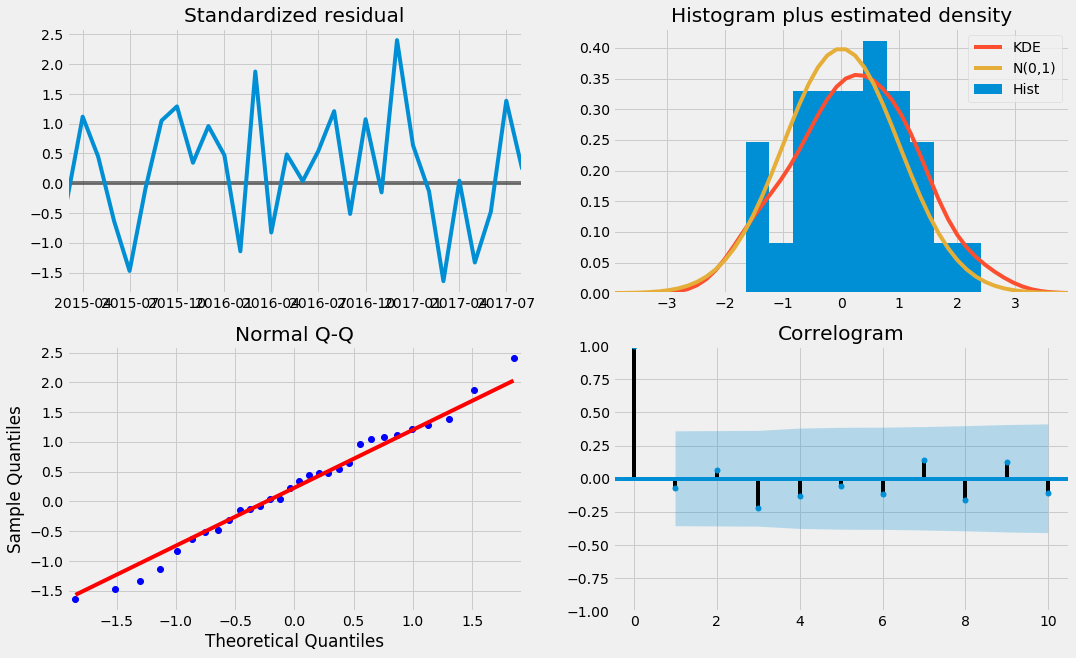First thing we have to look for is the residuals of our model.They have to be uncorrelated and normally distributed.

If it is not the case, we should further improve the model.

• The graph on the top right, the red KDE line follows closely with with the yellow line, the normal distribution line. This is an indication that the residuals may be normally distributed.
• The graph on the bottom left shows that the ordered distribution of residuals (blue dots). They follow the linear trend of the samples taken from a standard normal distribution with N(0, 1). Again, this is an indication that the residuals are normally distributed.

## Validating Forecasts¶

This is the time where we will use the model to predict future oil prices.

We will compare the predicted oil price with the real ones, which wil help us understand the accuracy of our forecasts

The attributes get_prediction() and conf_int() allow us to obtain the values and associated confidence intervals for forecasts of our dataset.

In :
# the code below requires the forecasts to start at June 2015.
# the dynamic=False argument ensures that we produce one-step ahead forecasts,
# meaning that forecasts at each point are generated using the full history up to that point.

pred = results.get_prediction(start=pd.to_datetime('2015-06-01'), dynamic=False)
pred_ci = pred.conf_int()

In :
ax = oil['2013':].plot(label='observed', figsize=(15, 6))

ax.fill_between(pred_ci.index,
pred_ci.iloc[:, 0],
pred_ci.iloc[:, 1], color='k', alpha=.2)

ax.set_xlabel('Date')
plt.legend()

plt.show()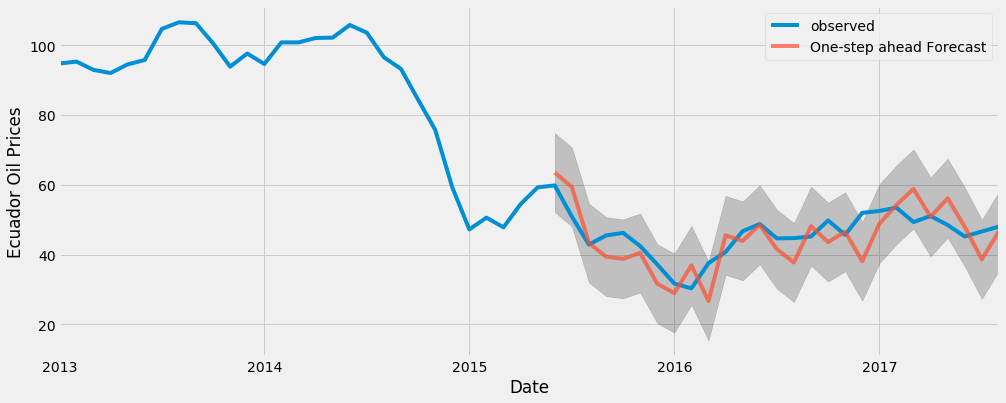The forecasts align with the true values well, but don't show any obvious trend.

We should now quantify the accuracy of our forecasts.

I will use the MSE (Mean Squared Error), that tell us the average error of our forecasts.

For each predicted value, we compute its distance to the true value and square the result.

Please note that we need to square the errors so that positive and negative values don't cabcel each other.

In :
y_forecasted = pred.predicted_mean
y_truth = oil['2015-06-01':]

# Compute the mean square error
mse = ((y_forecasted - y_truth) ** 2).mean()
print('The Mean Squared Error of our forecasts is {}'.format(round(mse, 2)))

The Mean Squared Error of our forecasts is 35.06


The MSE of our one-step ahead forecasts yields a value of 35.06, which is a little high.

But there is a better representation of our predictive results can be obtained using dynamic forecasts, that is, setting dynamic=True.

This means that we use information from the data up to a certain point and then the forecasts will be generated using values from previous forecasted values

In the code chunk below, we specify to start computing the dynamic forecasts and confidence intervals from June 2015 onwards.

In :
pred_dynamic = results.get_prediction(start=pd.to_datetime('2015-06-01'), dynamic=True)
pred_dynamic_ci = pred_dynamic.conf_int()

In :
ax = oil['2013':].plot(label='observed', figsize=(15, 6))
pred_dynamic.predicted_mean.plot(label='Dynamic Forecast', ax=ax)

ax.fill_between(pred_dynamic_ci.index,
pred_dynamic_ci.iloc[:, 0],
pred_dynamic_ci.iloc[:, 1], color='k', alpha=.25)

ax.fill_betweenx(ax.get_ylim(), pd.to_datetime('2015-01-01'), oil.index[-1],
alpha=.1, zorder=-1)

ax.set_xlabel('Date')

plt.legend()
plt.show()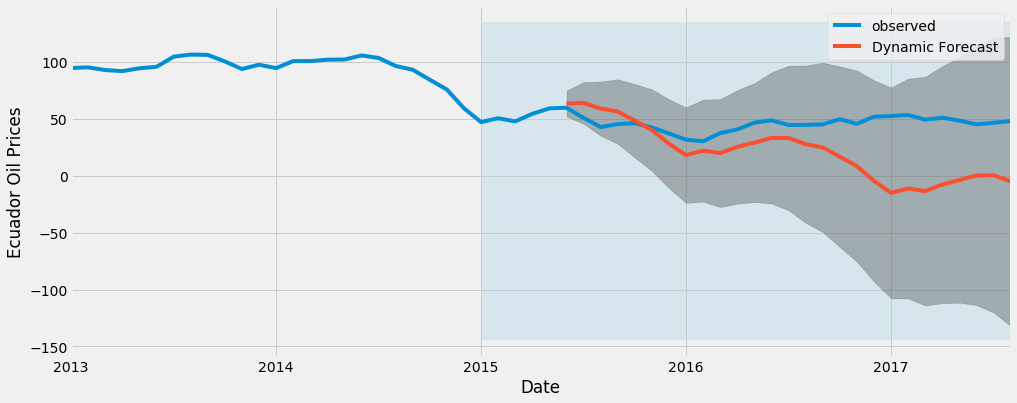Once again, we verify the performance of our forecasts by computing the MSE:

In :
# Extract the predicted and true values of our time series
y_forecasted = pred_dynamic.predicted_mean
y_truth = oil['2015-06-01':]

# Compute the mean square error
mse = ((y_forecasted - y_truth) ** 2).mean()
print('The Mean Squared Error of our forecasts is {}'.format(round(mse, 2)))

The Mean Squared Error of our forecasts is 1270.95


Here we are relying on less historical data than before, but even that, the MSE is high enough that at this point I should be thinking about applying a different model to predict oil prices.

Oil prices are a tricky thing to predict and the natural volatilty attached to it makes it hard to get a reasonable result.

## Producing and Visualizing Forecasts¶

I will finally describe how to forecast future value using Arima.

The get_forecast() attribute of our time series object can compute forecasted values for a specified number of steps ahead, in my case, 20 steps.

In :
# Get forecast 20 steps ahead in future
pred_uc = results.get_forecast(steps=20)

# Get confidence intervals of forecasts
pred_ci = pred_uc.conf_int()

In :
ax = oil.plot(label='observed', figsize=(15, 6))
pred_uc.predicted_mean.plot(ax=ax, label='Forecast')
ax.fill_between(pred_ci.index,
pred_ci.iloc[:, 0],
pred_ci.iloc[:, 1], color='k', alpha=.25)
ax.set_xlabel('Date')